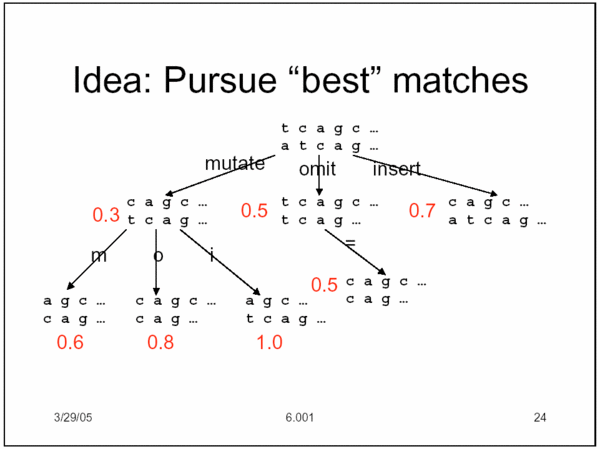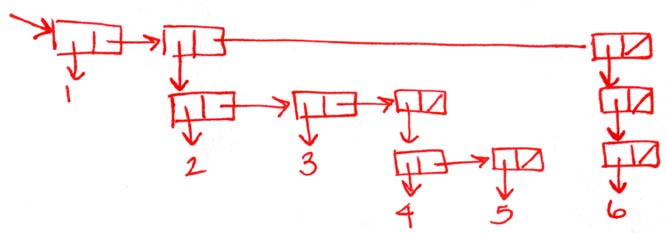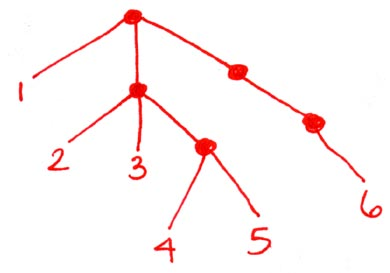```recitation 14: trees & search

---------------------------------------------------------------------------------------

* any questions on the project?

* any questions from lecture?

* depth-first search

* best-first search

* beam search

* tree vs. graph

* directed graph

* memoization

* what's the purpose of *search-debug* in the project?

* draw the stylized graph diagram for test-cycle:

(define test-cycle
(make-graph (list
(make-graph-element 'a '(b c) '(words for node a))
(make-graph-element 'b '(c) '(words for node b))
(make-graph-element 'c '(a) '(words for node c)))))---------------------------------------------------------------------------------------
(define my-tree (list 1 (list 2 3 (list 4 5)) (list (list 6))))

* draw the box & pointer diagram for my-tree* how does my-tree print?
(1 (2 3 (4 5)) ((6)))

* draw a "stylized tree diagram" for my-tree* how are they related?
Each node of the tree is a proper list in the box & pointer diagram.
If the list has n elements, the node has n branches.

(define (leaf? tree) (not (pair? tree)))

(define (tree-manip leaf-op init merge tree)
(if (null? tree)
init
(if (leaf? tree)
(leaf-op tree)
(merge (tree-manip leaf-op init merge (car tree))
(tree-manip leaf-op init merge (cdr tree))))))

* type of tree-manip: A->B, B, (B,B->B), tree<A> -> B

* use tree manip to square every element of a tree

(square-tree my-tree)  =>  (1 (4 9 (16 25)) ((36)))

(define (square-tree tree)
(tree-manip square nil cons tree))

* use tree-manip to compute the product of all the even-valued
leaves of the tree

(product-even my-tree)   =>  48

(define (product-even tree)
(tree-manip (lambda (x) (if (odd? x) 1 x)) 1 * tree))

* use tree-manip flatten a tree

(flatten my-tree)   =>   (1 2 3 4 5 6)

(define (flatten tree)
(tree-manip (lambda (x) (list x)) nil append tree))

* use tree-manip to "deep-reverse" a tree (reverse the order of
children at each node)

(deep-reverse my-tree)  =>  (((6)) ((5 4) 3 2) 1)

(define (deep-reverse tree)
(tree-manip
(lambda (x) x)
nil
(lambda (x y) (append y (list x)))
tree))

* use tree-manip to sum up the values of the leaves of the tree

(sum-leaves my-tree)  =>  21

(define (sum-leaves tree)
(tree-manip (lambda (x) x) 0 + tree))

* use tree-manip to create a new tree, which keeps the odd-valued
leaves of the original tree within the same tree structure, but
removes even valued leaves

(keep-odd-leaves my-tree) =>  (1 (3 (5)))
(keep-odd-leaves (list 1 2 3 4 5 6))  =>  (1 3 5)
(keep-odd-leaves (list 2 4 6))  =>  nil
(keep-odd-leaves (list (list 1) (list 4) (list 6))) =>  ((1))

(define (keep-odd-leaves tree)
(tree-manip
(lambda (x) (if (even? x) nil x))
nil
(lambda (x y) (if (null? x) y (cons x y)))
tree))

* for each of the above exercises, write down the types of arguments &
return value for the calls to tree-manip

---------------------------------------------------------------------------------------
```